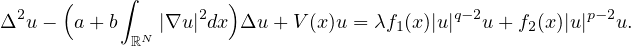Electron. J. Differential Equations, Vol. 2020 (2020), No. 44, pp. 1-15.

### Multiple positive solutions for biharmonic equation of Kirchhoff type involving concave-convex nonlinearities Fengjuan Meng, Fubao Zhang, Yuanyuan Zhang

Abstract:
In this article, we study the multiplicity of positive solutions for the biharmonic equation of Kirchhoff type involving concave-convex nonlinearities,Using the Nehari manifold, Ekeland variational principle, and the theory of Lagrange multipliers, we prove that there are at least two positive solutions, one of which is a positive ground state solution.

Submitted March 11, 2019. Published May 19, 2020.
Math Subject Classifications: 35J35, 35J40, 35J91.
Key Words: Biharmonic equation; ground state solution; Nehari manifold; concave-convex nonlinearity.

Show me the PDF file (370 KB), TEX file for this article.Fengjuan Meng School of Mathematics and Physics Jiangsu University of Technology Changzhou 213001, China email: fjmeng@jsut.edu.cn Fubao Zhang School of Mathematics Southeast University Nanjing 210096, China email: 101009933@seu.edu.cn Yuanyuan Zhang School of Business Jiangsu University of Technology Changzhou 213001, China email: zyylhh1227@163.com